# Know Month Name from the Given Date

By | May 12, 2016

Sage X3 provides an inbuilt function to retrieve the number and name of month from the particular date. Following are the functions you need to write for the same.

1. To retrieve number of month from given date:

month(exp_date)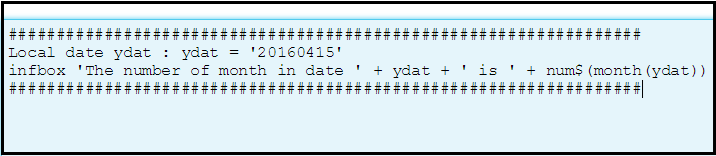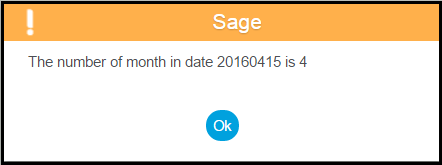1. To retrieve name of month from given date or given number of month:

month\$(exp_date)

month\$(exp_num)

Where,

exp_date          : date type expression

exp_num         : numerical expression

month\$            : extracts the name of the month for a valid date.

If the argument is numerical, it is a month number, calculated with modulo 12 (1 for “January”, etc.).

The result is a Char type.

You can pass either the date or number of a month as an argument to this function.

Refer the below screenshots better understanding.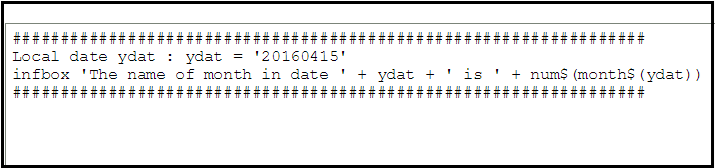Here, the date is passed as an argument to month\$() function.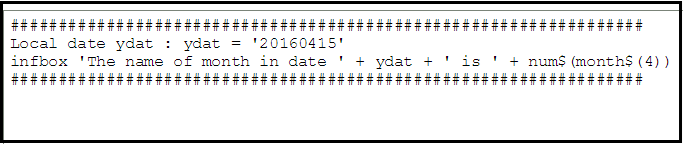Here, the number of a month is passed as an argument to month\$() function.

Output for above two snippets is: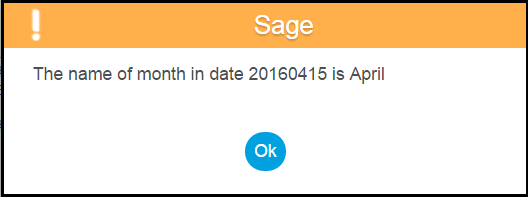Hope this blog helps!